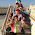# Hackerrank Chocolate Feast solution

The Solution for hackerrank problem, Chocolate Feast using C Program.
Passed Test cases: 9 out of 9

### SOURCE CODE:

`````` #include <stdio.h>
#include <string.h>
#include <math.h>
#include <stdlib.h>
int main() {
int t, n, c, m;
scanf("%d", &t);
while ( t-- )
{
scanf("%d%d%d",&n,&c,&m);
/** Write the code to compute the answer here. **/
{
do{
temp-=m;
}while(temp>=m);
}
}
return 0;
}
``````

1.Use % operator to minimize the iterations instead of buying one-one chocolate each time

2.#include
#include
#include
#include
#include
#include
#include
#include
#include
#include
#include
using namespace std;

int main(){
int t,n,c,m,w,o,e;
cin >> t;
while(t--){
o=0;
cin >> n >> c >> m;
w=n/c;
o=w;
do{
if(w>m)
e=w%m;//1,0
else
e=0;
o=o+w/m;//4
w=e+w/m;//2
}while(e&&(w>=m));

cout << o << endl;

}
return 0;
}
3.